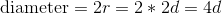## Example Questions

### Example Question #1 : How To Find The Diameter Of A Sphere

If a sphere has a volume of, what is its diameter?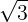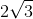Explanation:

1. Use the volume to find the radius: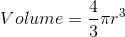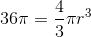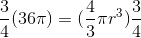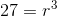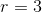2. Use the radius to find the diameter: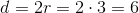### Example Question #2 : How To Find The Diameter Of A Sphere

A sphere has a volume of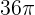. What is its diameter?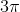Cannot be determined from the information givenExplanation:

This question relies on knowledge of the formula for volume of a sphere, which is as follows: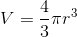In this equation, we have two variables,and. Additionally, we know that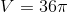andis unknown. You can begin by rearranging the volume equation so it is solved for, then plug inand solve for:

Rearranged form: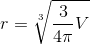Plug infor V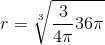Simplify the part under the cubed root

1) Cancel the's since they are in the numerator and denominator.

2) Simplify the fraction and the: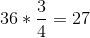Thus we are left with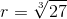Then, either use your calculator and enter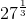Or recall that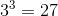in order to find that.

We're almost there, but we need to go a step further. Dodge the trap answer "" and carry on. Read the question carefully to see that we need the diameter, not the radius.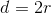So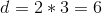is our final answer.

### Example Question #3 : How To Find The Diameter Of A Sphere

A spherical plastic ball has a diameter of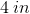. What is the volume of the ball to the nearest cubic inch?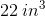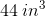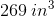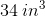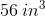Explanation:

To answer this question, we must calculate the volume of the ball using the equation for the volume of a sphere. The equation for the volume of a sphere is four-thirds multiplied by pi, which is then multiplied by the radius cubed. The equation can be written like this:We are given the diameter of the sphere in the problem, which is. To get the radius from the diameter, we divide the diameter by. So, for this data: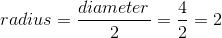We can then plug our newly found radius of two into the equation to find the volume. For this data: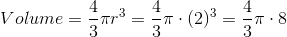We then multiplyby.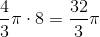We finally substitute 3.14 for pi and multiply again to get our answer.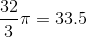The question asked us to round to the nearest whole cubic inch. To do this, we round a number up one place if the last digit is a 5, 6, 7, 8, or 9, and we round it down if the last digit is a 1, 2, 3, or 4. Therefore: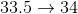Therefore our answer is.

### Example Question #4 : How To Find The Diameter Of A Sphere

A boulder breaks free on a slope and rolls downhill. It rolls for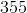complete revolutions before grinding to a halt. If the boulder has a volume of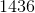cubic feet, how far in feet did the boulder roll? (Assume the boulder doesn't lose mass to friction). Roundto 3 significant digits. Round your final answer to the nearest integer.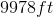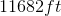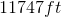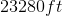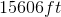Explanation:

The formula for the volume of a sphere is:To figure out how far the sphere rolled, we need to know the circumference, so we must first figure out radius. Solve the formula for volume in terms of radius: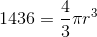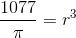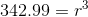Since the answer asks us to round to the nearest integer, we are safe to roundtoat this point.

To find circumference, we now apply our circumference formula: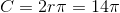If our boulder rolledtimes, it covered that many times its own circumference.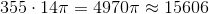Thus, our boulder rolled for### Example Question #5 : How To Find The Diameter Of A Sphere

Find the diameter of a sphere whose radius is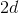.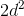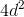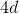To solve, simply remember that diameter is twice the radius. Don't be fooled when the radius is an algebraic expression and incorporates the arbitrary constant. Thus,